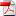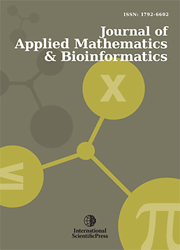# Journal of Applied Mathematics & Bioinformatics

#### Spectral relationships of Volterra- Fredholm integral equations in some domains

•[ Download ]
• Times downloaded: 1181
• Abstract

In this work, the solutions of Volterra- Fredholm integral equations of the first and second kind in one, two and three dimensional are obtained in the space L2 (Ω) × C [0,T] , T < 1. The Fredholm integral term is measured with respect to position, where Ω is the domain of integration; while Volterra integral is measured with respect to time. The solutions are obtained, using two different methods. For the first method, we have a Volterra integral equation, while for the second method, we obtain a linear system of Fredholm integral equation. Several spectral relationships are obtained when the kernel of position takes a logarithmic form, Carleman function, elliptic integral form, potential function, generalized potential function, Macdonald kernel, and other interesting cases are discussed.ISSN: 1792-6939 (Online)
1792-6602 (Print)